Trigonometry : Period and Amplitude

Example Questions

Example Question #1 : Period And Amplitude

What is the amplitude in the graph of the following equation: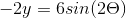Explanation:

The general form for a sine equation is: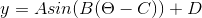The amplitude of a sine equation is the absolute value of.

Since our equation begins with, we would simplify the equation: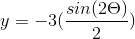The absolute value ofwould be.

Example Question #2 : Period And Amplitude

What is the amplitude of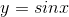Explanation:

Amplitude describes the distance from the middle of a periodic function to its local maximum.covers the range from -1 to 1. Thus, it covers a distance of 2 vertically. Half of this, or 1, gives us the amplitude of the function. It is often helpful to think of the amplitude of a periodic function as its "height".

Example Question #3 : Period And Amplitude

What is the amplitude of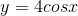Explanation:

The amplitude of a function describes its height from the midline to the maximum. The amplitude of the parent function,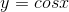, is 1, since it goes from -1 to 1. In this case our function has been multiplied by 4. Think of the effects this multiplication has on the outputs. In, we get our maximum at, and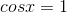. Here, we will get 4. The same thing happens for our minimum, at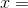,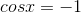. Here, we get -4. Thus, by this analysis, it is clear that the amplitude is 4. In the future, remember that the number preceding the cosine function will always be its amplitude.

Example Question #1 : Period And Amplitude

What is the period of the function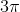Explanation:

By definition, the period of a function is the length offor which it repeats.starts at 0, continues to 1, goes back to 0, goes to -1, and then back to 0.

This complete cycle goes fromto.

Example Question #5 : Period And Amplitude

What is the period and amplitude of the following trigonometric function?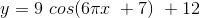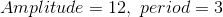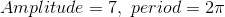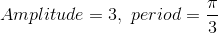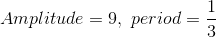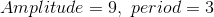Explanation:

Recall the form of a sinusoid: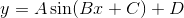or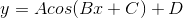The important quantities for this question are the amplitude, given by, and period given by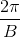.

For this problem, amplitude is equal to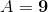and period is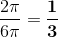.

Example Question #6 : Period And Amplitude

What is the period of the following function?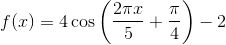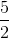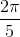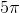Explanation:

The period of the standard cosine function is.

We can find the period of the given function by dividingby the coefficient in front of, which is: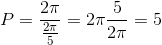.

Example Question #7 : Period And Amplitude

Write the equation of sine graph with amplitude 3 and period of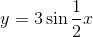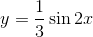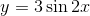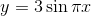None of the aboveExplanation:

Giving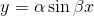,

where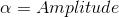and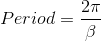Then,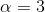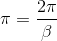,

hence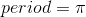.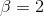.

Therefore,Example Question #8 : Period And Amplitude

Which of the given functions has the greatest amplitude?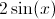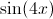The amplitude of a function is the amount by which the graph of the function travels above and below its midline. When graphing a sine function, the value of the amplitude is equivalent to the value of the coefficient of the sine. Similarly, the coefficient associated with the x-value is related to the function's period. The largest coefficient associated with the sine in the provided functions is 2; therefore the correct answer is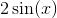.